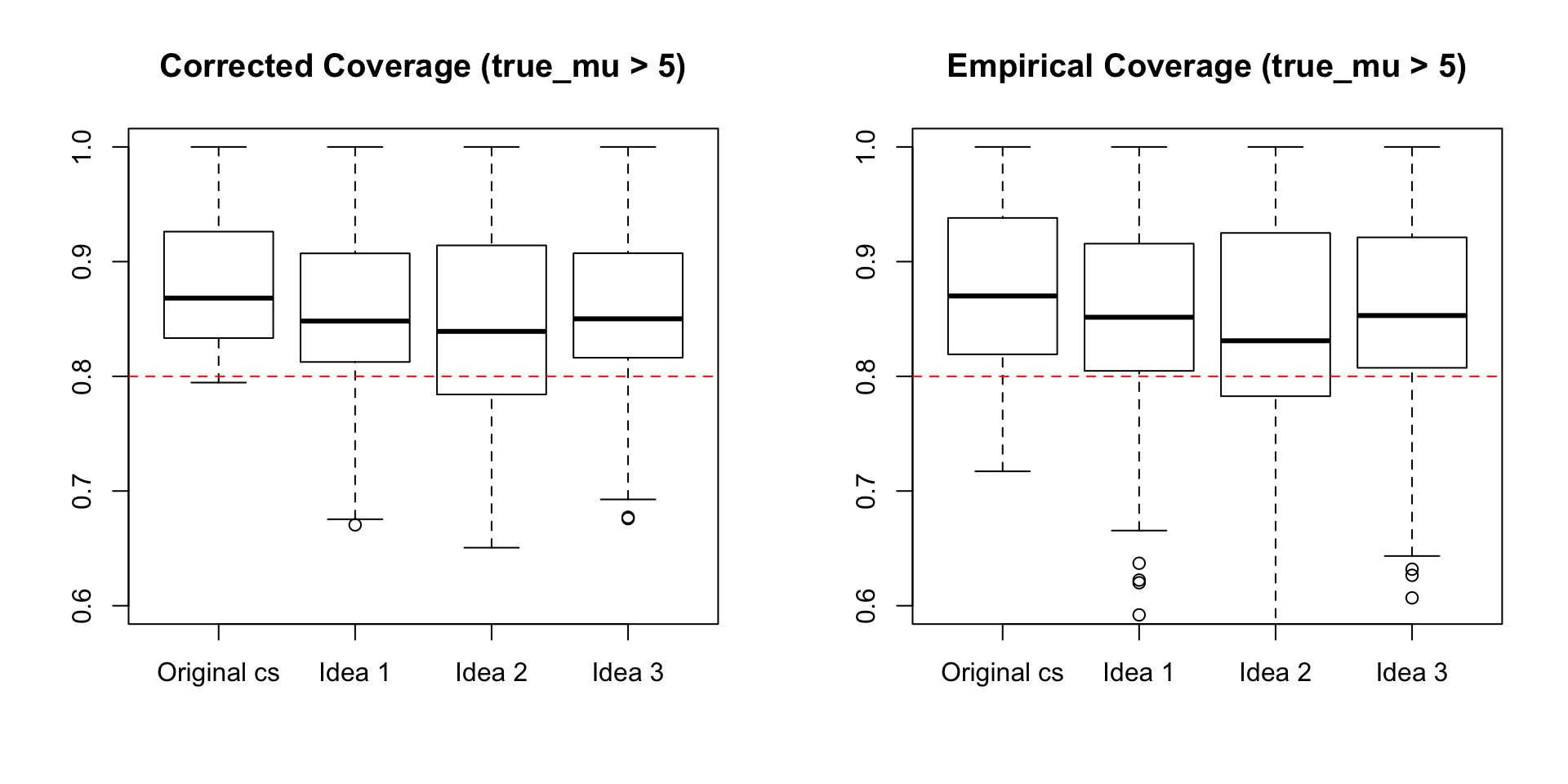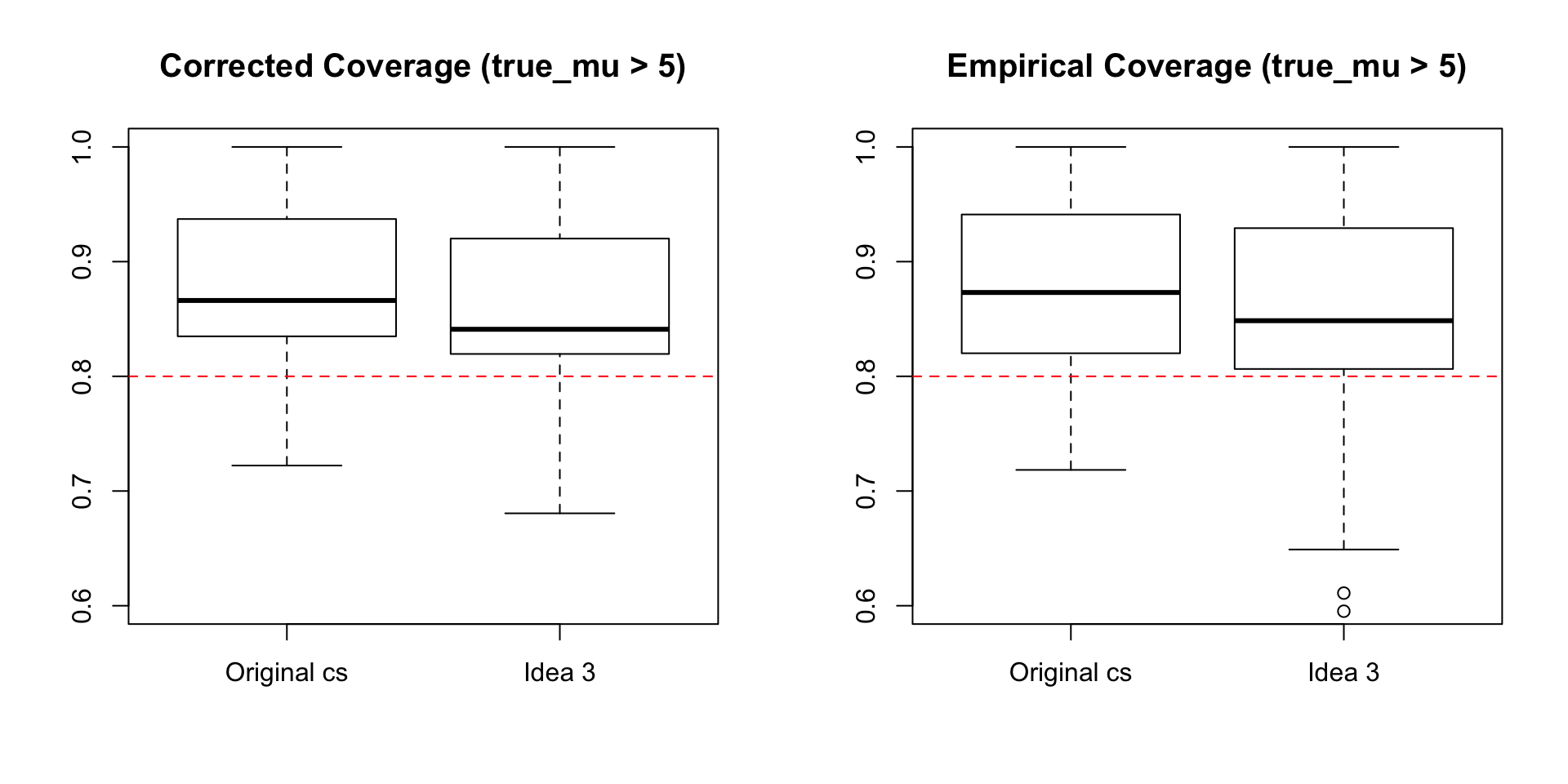### Obtain Required Threshold

Aim: Obtain a required threshold ($$x$$) from a desired coverage ($$y$$).

Idea 1: Obtain predicted threshold from each GAM (logit(covered)~logit(cumsum)), normalise each with pp and sum

Idea 2: Predict $$y$$ for $$x \in (0,1)$$ from each GAM, average these and use these to predict required threshold

Idea 3: As above but normalise each with pp• Plots above show that the methods do bring the coverage of the new credible sets closer to 0.8. Idea 2 can be ruled out as it results in too many credible sets with coverage below that desired.

• Idea 3 only:• Idea 3 seems to work well at obtaining new credible sets with closer to the required coveraged of the true causal variant. Is there a way to not use GAMs at all? Idea 4 investigates this.

### Idea 4

• Apply correction factor repeatedly with different thresholds to find the required threshold to obtain the desired corrected coverage.

• The slow step is simulating posterior probabilities. This does not need to be done everytime we call the function. Infact we now simulate pps FIRST and then input them (list of matrices) into the quick_corrcov function along with the threshold and the original posterior probabilities. This function uses C++ credsetmat function to quickly form credible sets and finds the proportion of these containing the CV, weighted by posterior probabilities. This is VERY quick (~4 secs for 1000 simulations for each snp considered causal = 2e+05 credible sets).

The quick_corrcov function takes approximately 4 seconds to report the corrected coverage estimate of the credible set, using a specified threshold.

## "if you use this threshold, the resultant credible set has this corrected coverage"

quick_corrcov <- function(thr, simulated.pps, pp){
n_pps <- length(simulated.pps)
args <- 1:nsnps

d5 <- lapply(1:n_pps, function(x) {
credset3(simulated.pps[[x]], CV = rep(args[x], dim(simulated.pps[[x]])), thr = thr)
})

prop_cov <- lapply(d5, pred_na) %>% unlist()
sum(prop_cov * pp)
}
• This can be further developed to also provide the credible set obtained using this threshold.

The quick_corrcov_cs function takes approximately 4 seconds to report a list of the corrected coverage estimate, the new credible set, the threshold used and the size.

## "if you use this threshold, the resultant credible set is this and has this corrected coverage"

quick_corrcov_cs <- function(thr, simulated.pps, pp){
n_pps <- length(simulated.pps)
args <- 1:nsnps

d5 <- lapply(1:n_pps, function(x) {
credset3(simulated.pps[[x]], CV = rep(args[x], dim(simulated.pps[[x]])), thr = thr)
})

prop_cov <- lapply(d5, pred_na) %>% unlist()
corr_cov <- sum(prop_cov * pp)

o <- order(pp, decreasing = TRUE)
cumpp <- cumsum(pp[o])
wh <- which(cumpp > thr)
list(credset = names(pp)[o[1:wh]], corr.cov = corr_cov, thr = thr, size = cumpp[wh])
}

### Idea 4 code

#### 1. Vary threshold

• BUT the aim of all of these ‘ideas’ is to provide the user with the threshold they should be using to obtain a credible set with the desired coverage. Of course, they could keep using quick_corrcov_cs above until the corrected coverage is where they want it to be, but we need to automate this.

• Could use the following function to obtain a credible set with corrected coverage within some accuracy of the desired coverage. This uses C++ code to generate the credible sets from the simulated pps, do we really need to transfer the whole thing to C? Need to decide when to break the function - i.e. if it is impossible to get within this accuracy as there is one CV holding all the pp - include counter (this doesn’t seem to be stopping after 4 goes??)

new_cs <- function(desired.cov, acc = 0.05, simulated.pps, pp){

corr.cov <- quick_corrcov(desired.cov, simulated.pps, pp)
is_within_tolerance <- FALSE
thr1 <- desired.cov
counter <- 1

while (counter < 4 | !is_within_tolerance) {
if(dplyr::between(corr.cov, desired.cov, desired.cov+acc)){
is_within_tolerance <- TRUE
}

# if corr.cov is too high
if(corr.cov > desired.cov+acc){ # then run again with lower threshold (remove variants)
thr1 <- thr1 - (desired.cov-corr.cov)
corr.cov <- quick_corrcov(thr1, simulated.pps, pp)
next
}

# if corr.cov is too low
if(corr.cov < desired.cov){ # then run again with higher threshold (add variants)
thr1 <- thr1 + (desired.cov-corr.cov)
corr.cov <- quick_corrcov(thr1, simulated.pps, pp)
next
}
counter <- counter + 1
}

o <- order(pp, decreasing = TRUE)  # order index for true pp
cumpp <- cumsum(pp[o])  # cum sums of ordered pps
wh <- which(cumpp > thr1)  # how many needed to exceed thr
list(credset = o[1:wh], corr.cov = corr.cov, thr = thr1, counter=counter)
}

new_cs(0.8, 0.05, pps, pp0)

• A better (more robust) approach is the method below, which systematically removes/adds variants from the credible set until we have a new credible set with corrected coverage within some accuracy.

• Odd scenarios I had to consider:

1. Only 1 variant in the set (so can’t get a smaller credible set)
2. Alternating endlessly between over desired.cov+accuracy and below desired.cov
desiredcov_cs <- function(desired.cov, acc = 0.05, simulated.pps, pp){
n_pps <- length(simulated.pps)
args <- 1:length(pp)
thr <- desired.cov

d5 <- lapply(1:n_pps, function(x) {
credset3(simulated.pps[[x]], CV = rep(args[x], dim(simulated.pps[[x]])), thr = thr)
})

prop_cov <- lapply(d5, pred_na) %>% unlist()
corr_cov <- sum(prop_cov * pp)

o <- order(pp, decreasing = TRUE)
cumpp <- cumsum(pp[o])
wh <- which(cumpp > thr)

is_within_tolerance <- FALSE

while (!is_within_tolerance) {
if(dplyr::between(corr_cov, desired.cov, desired.cov+acc)){
is_within_tolerance <- TRUE
}

if(wh==1){
warning("Only 1 variant in the credible set, so can't make it any smaller")
is_within_tolerance <- TRUE
}

# if corr.cov is too high
if(!wh==1 & corr_cov > desired.cov+acc){
wh <- wh-1 # remove a variant from the cs
thr <- cumsum(pp[o][1:wh-1])[wh-1]+(cumsum(pp[o][1:wh])[wh]-cumsum(pp[o][1:wh-1])[wh-1])/2 # new thr required
corr_cov <- quick_corrcov(thr, simulated.pps, pp)
next
}

# if corr.cov is too low
if(!wh==1 & corr_cov < desired.cov){
wh <- wh+1 # add a variant to the cs
thr <- cumsum(pp[o][1:wh-1])[wh-1]+(cumsum(pp[o][1:wh])[wh]-cumsum(pp[o][1:wh-1])[wh-1])/2 # new thr required
corr_cov <- quick_corrcov(thr, simulated.pps, pp)

if(corr_cov > desired.cov+acc){
warning("This is the smallest credible set with corrected coverage above the desired coverage")
is_within_tolerance <- TRUE
}
next
}
}
list(credset = names(pp)[o[1:wh]], corr_cov = corr_cov, thr = thr, size = cumsum(pp[o])[wh], nvar = wh)
}
• I’ve tried this with several datasets and it looks really promising - very quick and accurate.

• Note that this function can be used to find the smallest credible set possible with corrected coverage greater than the desired coverage by setting acc to a very small value.

### Can we use fewer replicates in the correction?

• Last week I compared nrep=1000, 5000, 10K and the results were pretty much the same. Can we use even fewer reps?